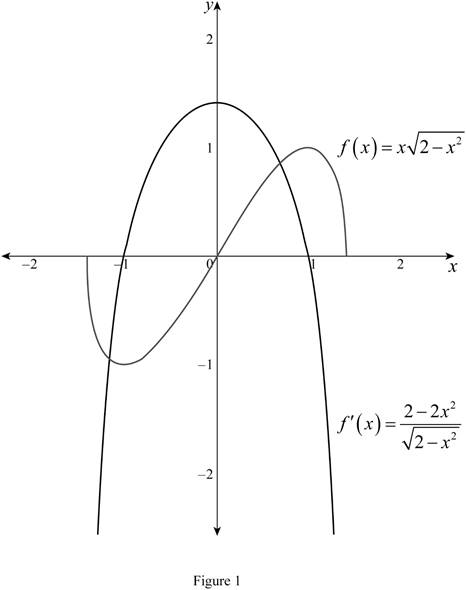# The derivative of f ( x ) = x 2 − x 2 .### Single Variable Calculus: Concepts...

4th Edition
James Stewart
Publisher: Cengage Learning
ISBN: 9781337687805### Single Variable Calculus: Concepts...

4th Edition
James Stewart
Publisher: Cengage Learning
ISBN: 9781337687805

#### Solutions

Chapter 3.4, Problem 47E

(a)

To determine

## To find: The derivative of f(x)=x2−x2.

Expert Solution

The derivative of f(x)=x2x2 is f(x)=22x22x2_.

### Explanation of Solution

Given:

The function is f(x)=x2x2.

Result used:

The Power Rule combined with the Chain Rule:

If n is any real number and g(x) is differentiable function, then

ddx[g(x)]n=n[g(x)]n1g(x) (1)

Derivative Rule: Product Rule:

If f(x). and g(x) are both differentiable function, then

ddx[f(x)g(x)]=f(x)ddx[g(x)]+g(x)ddx[f(x)] (2)

Calculation:

Obtain the derivative of f(x).

f(x)=ddx(f(x))=ddx(x2x2)

Apply the product rule as shown in equation (2),

f(x)=xddx[2x2]+2x2ddx[x]=xddx(2x2)12+2x2[1x11]=xddx(2x2)12+2x2[x0]=xddx(2x2)12+2x2

Apply the power rule combined with the chain rule as shown in equation (1),

f(x)=x12(2x2)121ddx(2x2)+2x2=x12(2x2)122(ddx(2)ddx(x2))+2x2=x12(2x2)12(0(2x21))+2x2=x12(2x2)12(2x1)+2x2

Simplify further and obtain the derivative,

f(x)=x22x2+2x2=x2+2x22x22x2=x2+2x22x2=22x22x2

Therefore, the derivative of f(x)=x2x2 is f(x)=2(1x2)2x2_.

(b)

To determine

Expert Solution

### Explanation of Solution

Given:

The function is f(x)=x2x2.

The derivative of f(x) is 22x22x2.

Graph:

Use the online graphing calculator and draw the graph of the functions as shown below in Figure 1.From Figure 1 it is observe that,

If f(x) is positive then f(x) is increasing function.

If f(x) is negative then f(x) is decreasing function.

If f(x) is local extrema (that is local minimum or local maximum), then f(x) is crosses the x axis (f(x)=0).

Hence, the function f(x) is reasonable.

### Have a homework question?

Subscribe to bartleby learn! Ask subject matter experts 30 homework questions each month. Plus, you’ll have access to millions of step-by-step textbook answers!